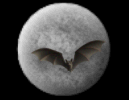God & Science Forum Message Forums: Atm · Astrophotography · Blackholes · Blackholes2 · CCD · Celestron · Domes · Education Eyepieces · Meade · Misc. · God and Science · SETI · Software · UFO · XEphemBe the first pioneers to continue the Astronomy Discussions at our new Astronomy meeting place...The Space and Astronomy Agora A Definition Of Mathematical Truth Forum List | Follow Ups | Post Message | Back to Thread Topics | In Response ToPosted by Harvey on July 19, 2003 14:32:49 UTC

Not to beat a dead horse, but I got to thinking what a definition of mathematical truth would look like. The obvious candidate is to use Tarski's schema T or convention T:

"S is true if, and only if, p"

'S' is the name of the sentence, and 'p' is the proposition that the sentence refers to as 'true'. For example:

"snow is white", if , and only if, snow is white

The truth of "snow is white" is a true statement if the state of affairs of "snow is white" is obtained by snow actually being white.

So, if we take the popular T schema, we can use it for a mathematical definition of truth such as:

MT1: "2+2=4", if, and only if, 2+2=4

But, T schema is not a true definition of truth because it has no quantifiers. If we add some quantifiers:

MT1': "2+2=4", if, and only if, "2+2=4" actually expresses 2+2=4 in reality, and 2+2=4 actually obtains in reality

Okay, we've quantified what we mean by how "2+2=4" can be 2+2=4 and how 2+2=4 can be actually true, but the ugly phrases 'expresses in reality' and 'obtains in reality' come up in MT1'. Therefore, we need to eliminate this in favor of some mathematical abstract consideration which doesn't require matching reality with the mathematical abstraction. So, let's revise it:

MT2: "2+2=4", if, and only if, "2+2=4" actually expresses 2+2=4 as a mathematical abstract reality, and 2+2=4 actually obtains in this mathematical abstract reality.

So, MT2 seems to be getting closer to a proper mathematical definition of truth, but we still haven't defined what is meant by 'mathematical abstract reality' and what it means to express or obtain such in a mathematical abstract reality. Here is the real ball of wax. However we go about specifying this we are bound to be in for questions which we cannot properly answer. For example:

"object X lies on plane P", if, and only if, "object X lies on plane P" actually expresses an object X lies on plane P as a mathematical abstract reality, and object X lies on plane P obtains in this mathematical abstract reality.

We have no way to specify what it means for an abstract object to 'lie' on a plane. Does this mean they touch? If they touch, then what touches? The object and plane are abstract and defining something as touching has no clear descriptive meaning. Similarly, where does this occur? In mathematical abstract reality? Who's mathematical abstract reality? Is everyone's reality the same as everyone else's abstact reality?

This might seem unfair to throw these kind of issues in the middle of a simple mathematical attempt to set-up an abstract situation, however this is the result of trying to establish a definition of mathematical truth if one chooses to do so.

I believe Hilbert was one of the first well-known mathematicians to recognize the folly of defining certain undefined terms, and in his axiomization of geometry unlike Euclid he didn't bother with the definitions of all of his terms. Rather, he simply supplied such terms as undefined. Instead, he focused on the conditions on how to treat and build on those terms, and modern axiomization was born. Truth in mathematics remains undefined, but the conditions of truth (truth functionals) is of course the heart and soul of mathematics and logic.